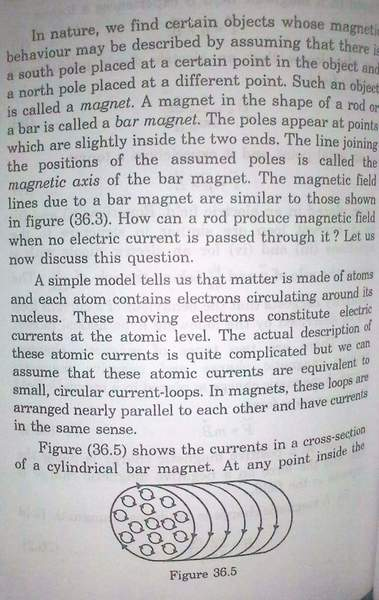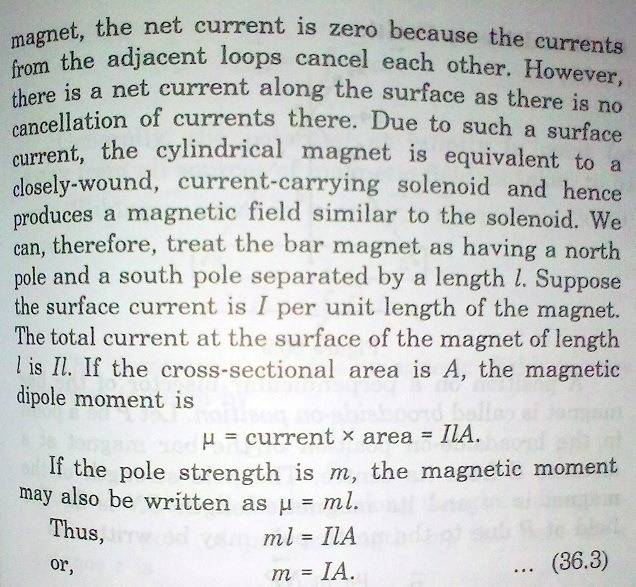# A basic question on Electromagnetic Induction

Abhishekdas

## Homework Statement

There is a circular loop in the horizontal plane and a bar magnet (with its north pole downwards) Is falling down along the axis of the loop.....
Now is the acceleration of the bar magnet less than,greater than or equal to g?

## The Attempt at a Solution

The explaination in the book is given as: The side of the loop facing the bar magnet is getting a North Polarity (this is because the direction ofcurrent is antclockwise from top by Lenz's Law). Now like poles repel and hence the bar magnet's acceleration is less than g.....

Firstly im not totally convinced by this concept . I mean i know like poles repel from a young age but at this stage i would like to know how exactly it happens(like in electrostatics Coulumb's Law gives the dir of forces which proves that like charges repel) and it would be great is someone explains me that.

Secondly i was trying to figure this out with the concept of force due to a magnetic field....But i cant do so because in a bar magnet where is tha charge? and what is the velocity? So basically I am clueless.....So guys help me out.....
I've attached a diagram for the sum if you need it.....

Thank you.....

## The Attempt at a Solution

#### Attachments

• curr.bmp
280.7 KB · Views: 512
Last edited:

Staff Emeritus
Homework Helper

## The Attempt at a Solution

The explaination in the book is given as: The side of the loop facing the bar magnet is getting a North Polarity (this is because the direction ofcurrent is antclockwise from top by Lenz's Law). Now like poles repel and hence the bar magnet's acceleration is less than g.....

Firstly im not totally convinced by this concept . I mean i know like poles repel from a young age but at this stage i would like to know how exactly it happens(like in electrostatics Coulumb's Law gives the dir of forces which proves that like charges repel) and it would be great is someone explains me that.
Okay, how about the fact that parallel wires attract each other when their currents are in the same direction, and repel if the currents are in the opposite direction? If you can accept that as an observed, experimental fact, then I offer the following explanation:

Imagine two equal loops, one above the other, and their planes are parallel to each other. Also, imagine that each has a current, but the currents run in opposite directions for the two loops. Since the currents are in opposite directions, the loops will repel each other, just as two parallel wires repel when their currents are in opposite directions.

But, the opposite currents means that one loop has its N pole upward, and the other has its N pole downward. So the loops have like poles pointing at each other. And we already know that the loops repel, so ... end of story.

Secondly i was trying to figure this out with the concept of force due to a magnetic field....But i cant do so because in a bar magnet where is tha charge? and what is the velocity? So basically I am clueless.....So guys help me out.....
I've attached a diagram for the sum if you need it.....
The charge is in the protons and electrons of the atoms. We don't know "the velocity", but it is primarily the motion of the electrons that gives rise to the magnetic field.

cupid.callin
Okay, how about the fact that parallel wires attract each other when their currents are in the same direction, and repel if the currents are in the opposite direction? If you can accept that as an observed, experimental fact, then I offer the following explanation:

Imagine two equal loops, one above the other, and their planes are parallel to each other. Also, imagine that each has a current, but the currents run in opposite directions for the two loops. Since the currents are in opposite directions, the loops will repel each other, just as two parallel wires repel when their currents are in opposite directions.

But, the opposite currents means that one loop has its N pole upward, and the other has its N pole downward. So the loops have like poles pointing at each other. And we already know that the loops repel, so ... end of story.

and you can easily prove how parallel currents in same direction attract using right hand ruld or cross product rule

Abhishekdas
Hi Redbelly98.....
The explaination which you gave does convince me about two loops but i exactly dont know how poles are created in bar magnets....Is it the same kind of thing...are there rectangular loops or something in bar magnets too? I google about "how poles are created in bar magnets" but didnt get any convincing answer as such.....So maybe you ca help me out there.....

And hi cupid.callin....
I know how to prove that parallel current carrying wires attract or repel depending on the direction of current....but here in case of a bar magnet as i mentioned above i dont know how poles are created in magnets.....So i dont know how to go about finding the force due to the magnetic field....

Anyway thank you both...expecting a reply soon.....

cupid.callin#### Attachments

Abhishekdas
Hey cupid.callin thanks a lot.....
this was of great help and this kind of a text was exctly what i was looking for.....The pole creations in a bar magnet.....Now i applied cross product rule and got my answer about how they repel.....really thank you so much.....